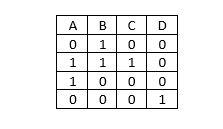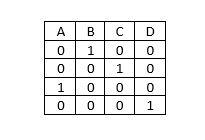# Amit Kumar Ojha

## Activity

• To your first modification of the 2**2 factorial design.  What you said you were doing and what you did are two entirely different things.  If you are going to set C = (0,0) and D = (1,1)  where the combinations are for A and B for those particular experiments then the actual design is:What you have done is this:This is just a standard ma…[Read more]

• @[email protected] – One of my pet gripes is the fact that when the folks were developing their terminology for six sigma the took the word “sigma” and applied it to the calculation indicated in my first post.  That formula is the formula for the sigma level which has nothing to do with the sigma of standard deviation fame.  Unfortunately, a n…[Read more]

• You might want to check the news letter option for this site.  As I understand it that is what the newsletter does.  Try contacting the website for additional details. 9 months, 4 weeks ago

• …one additional thought.  With measurements in parts per billion/trillion you are sure to run up against round-off error in whatever analysis program you are using.  This will be true even if you have (as most programs do today) double precision.  I would recommend you express the measures in scientific notation, drop the 10’s power and run th…[Read more]

• Cpk = minimum (USL-mean, mean – LSL)/(3*std)

Therefore, in order for Cpk to be negative your mean will have to either be less than the LSL or greater than the USL.

Sigma = minimum (USL-mean, mean – LSL)/std

Therefore, if the mean is greater than USL then (USL – mean) will be the smaller value and it will be negative which means Sigma will…[Read more]

1. From Agresti Categorical Data Analysis 2nd Edition page 3

” Variables are classified as continuous or discrete, according to the number of values they can take. Actual measurements of all variables occurs in a discrete manner, due to precision limitations in…[Read more]

• It sounds like you are taking some kind of exam or course or something and trying to match whatever is offered on a multiple choice problem – this is fine but there is something you should keep in mind when you are faced with questions like this in the real world and that is the issue of significant digits.

It is possible to get measurements out…[Read more]

• Obviously the problem is assuming a normal distribution of the data.

The basic formula for Cp in this case is

Cp = (USL-LSL/(6*std)

So, LSL = 330, USL = 330+10.3 and you want a minimum of 1.33 for Cp – therefore plug in the numbers in the above equation, re-arrange and solve for std. 10 months, 2 weeks ago

• I agree with @Straydog – the issue is that of statistics.  If we assume you have a mathematical background that includes algebra and if, for whatever reason,you can’t take a course in basic statistics then I would recommend working your way through the following books:

1. A Cartoon Guide to Statistics – Gonick and Smith – I’ve recommended this…[Read more]

• Things are fine on my end @cseider – hope all is well with you too.  As for the homework – it’s pretty quiet around these parts and I was feeling generous…    :-) 11 months ago

• It’s just the sums of the squares of the deviation from the mean divided by the total count minus 1.

So, for a single item the average is  (10 + 0)/2 = 5

The deviations from the mean are

10 – 5 = 5

0-5 = -5

The squares of those deviations are

5^2 = 25

(-5)^2 = 25

there are two observations so  2 – 1 = 1

Therefore the variance per item i…[Read more]

• I’m not sure where we’re going with this discussion.  The focus of your initial post was that of coil rejection due to defects.  In the follow up discussion I was left with the impression defect reduction and thus increased acceptance of coils was your main concern.  I was also left with the impression that one factor known be a contributor to de…[Read more]

• I’ve been giving your problem some thought and I can’t think of any single statistic that will capture consistency both with respect to stability of system variability and constancy of trending.  The issue you are facing is that of an evolution of both variation and trend across time. As far as I know, if time is involved you will have to take it…[Read more]

• Based on your post there not only does not seem to be any need for a 1-10 rating but, it doesn’t sound like your colleague has any idea of how to build a meaningful 1-10 rating scale that would be of any value to you.

What your post does suggest is you have some understanding of why coils are rejected.  For example, you mentioned dark surface on…[Read more]

• The question you are asking is one of a comparison of two proportions.  Specifically you want to know if the difference between 100% and 82.5% is statistically significant for a sample of 315.

The key is to recognize that the proportions are 315/315 and 260/315.  In other words, with a baseline of 315 in both cases we have a situation where t…[Read more]

• The short answer to you second post is: there’s nothing like that out there.

Let’s take another look at what you are asking:

You said: “I was looking for some current affairs metric that can tell me if something like the historical average growth rate will continue or if a certain ratio will stay stable in the future.”

If a measurement such as…[Read more]

• I might be missing something but, as written, your post appears to be expressing two different requirements.  You say you want to predict variation, specifically predict variation trending, however, the examples you give have nothing to do with prediction.  Both both CD and CV are strictly summations of current affairs – they don’t have any p…[Read more]

• ….an additional thought.  I was going over the case study and looking at the data set for Case #2 and it occurred to me that, if you want to, you can use that data set as a starting point for what would amount to an excellent self teaching exercise.

In the attachment I’ve rearranged the data to make the composite nature of the design more…[Read more]

• The way you would determine the minimum and maximum settings shown on page 28 would be to take the regression models for collapse and burst pressure and put them in an optimizing program and run them.  I don’t know Minitab but I do know it has such an option.

The problem is the author has not provided the models he generated using the data.  S…[Read more]

• I might be missing something but my understanding of the scenario you described is as follows:

a. Quality team takes a 10% sample of the total output.  With a total output of 1000 this becomes a sample of 100.

b. Quality team does a 100% inspection of the 100 samples and finds 10 defects in that sample.  Therefore, their claim, based on the e…[Read more]